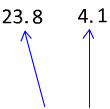# Multiplying Decimals

To multiply decimals we use a different set of steps than we would use to add or subtract decimals. In fact, you do not have to line up the decimals at all.

Ex. 1) 23.8 x 4.1

To solve this problem, we will first pretend that there are no decimals at all. Start by solving the question 238 x 41.So we could estimate that the answer should be around 20 x 4 = 80. That would mean that the decimal should go after the 97, to give us an answer of 97.58.

Therefore, 23.8 x 4.1 = 97.58.

However, there is a rule that you can use to know where to put the decimal without having to estimate.

Take a look at how many numbers come after the decimal point in each of the numbers we multiplied.There is one digit after each of the decimal points, making two numbers total. This tells us that there needs to be two numbers after the decimal point in our answer as well.

Ex. 2) 16.903 x 2.2

Start by multiplying 16903 x 22Now, take a look at how many digits are after the decimal points.Therefore, 16.903 x 2.2 = 37.1866

Let's check our answer using estimation:

20 x 2 = 40 We can see from our estimation that 37.1866 is a reasonable answer.

Let's go over the steps to multiply decimals.

Step 1: Multiply the numbers and ignore the decimal points.

Step 2: Count up how many number of digits that come after the decimal points in both factors.

Step 3: Place the decimal in the product so that the same number of digits comes after the decimal point in the answer.